# A beam with a square cross-section is in a combined state of loading. There is an axial force N 350 kN acting in the x-...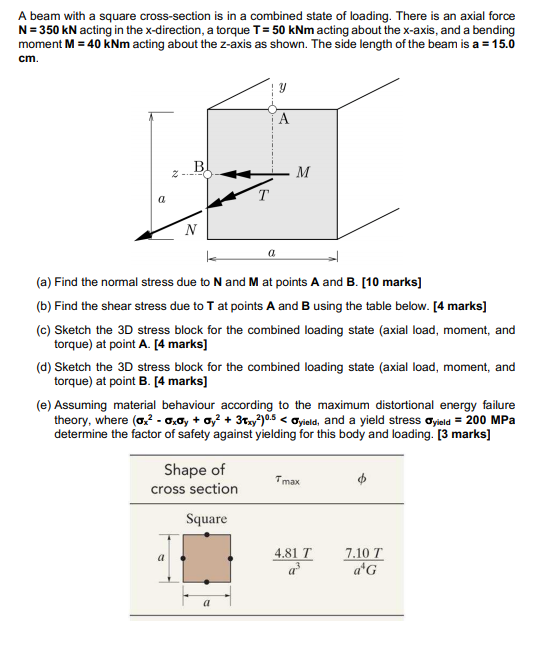A beam with a square cross-section is in a combined state of loading. There is an axial force N 350 kN acting in the x-direction, a torque T 50 kNm acting about the x-axis, and a bending moment M 40 kNm acting about the z-axis as shown. The side length of the beam is a 15.0 cm. B T a (a) Find the nomal stress due to N and M at points A and B. [10 marks] (b) Find the shear stress due to T at points A and B using the table below. [4 marks] (c) Sketch the 3D stress block for the combined loading state (axial load, moment, and torque) at point A. [4 marks] (d) Sketch the 3D stress block for the combined loading state (axial load, moment, and torque) at point B. [4 marks] (e) Assuming material behaviour according to the maximum distortional energy failure theory, where (ao2 - aa, + a,^+ 3y05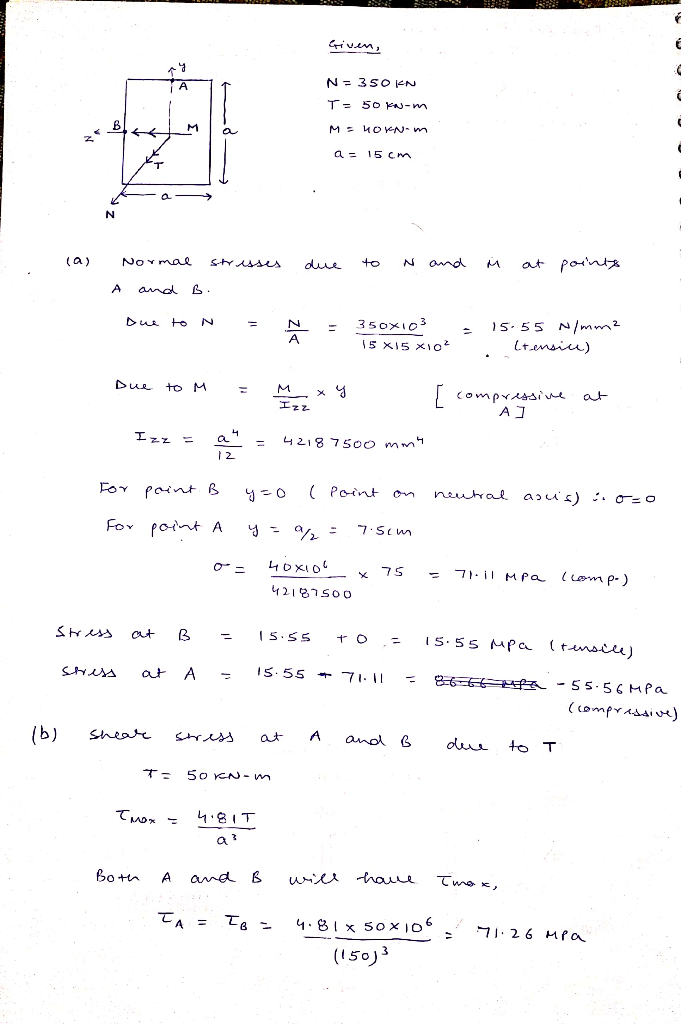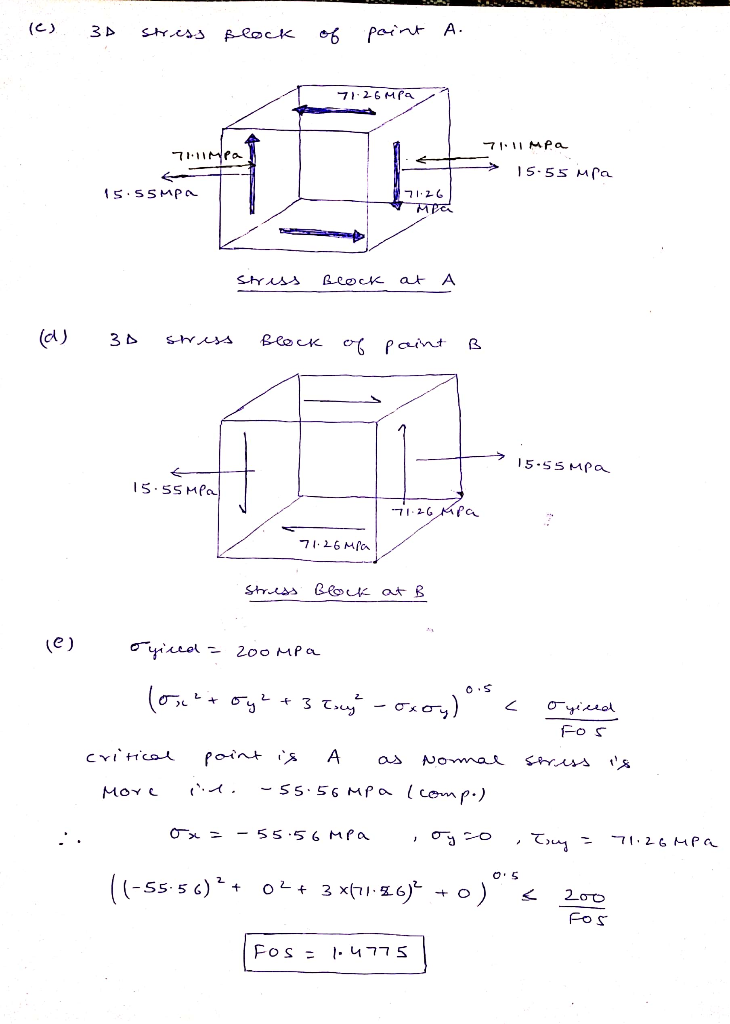##### Add Answer of: A beam with a square cross-section is in a combined state of loading. There is an axial force N 350 kN acting in the x-...
Similar Homework Help Questions
• ### As shown in Figure 8, the structural member (beam) is 7m long, carries a 2 kN point load, a 1.2 kN/m uniformly distribu...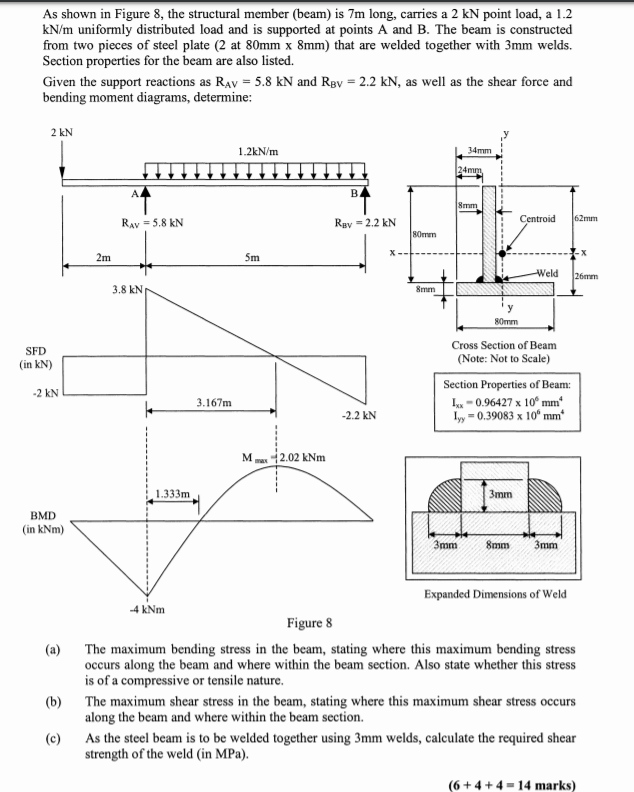As shown in Figure 8, the structural member (beam) is 7m long, carries a 2 kN point load, a 1.2 kN/m uniformly distributed load and is supported at points A and B. The beam is constructed from two pieces of steel plate (2 at 80mm x 8mm) that are welded together with 3mm welds. Section properties for the beam are also listed. Given the support reactions as RAv 5.8 kN and RBv 2.2 kN, as well as the shear force...

• ### 1. A beam has a max moment of 45 kN-m. The cross section of the beam is shown in the figure below...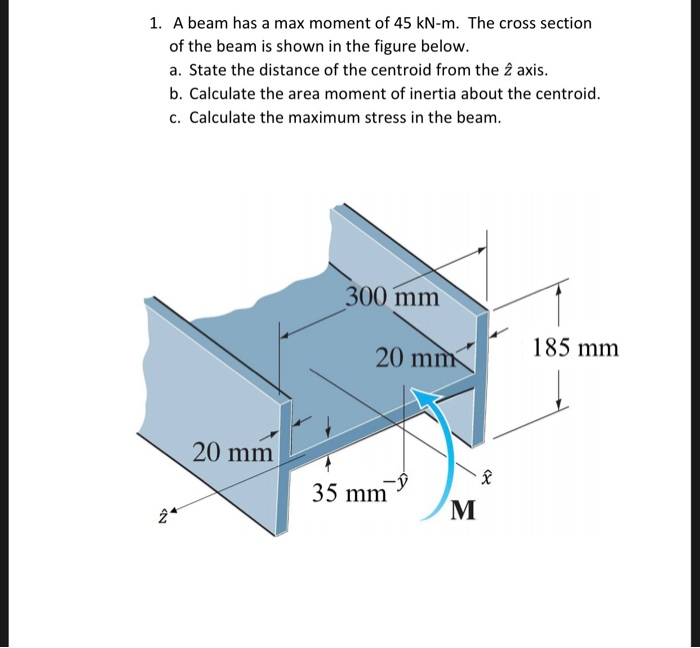1. A beam has a max moment of 45 kN-m. The cross section of the beam is shown in the figure below. a. State the distance of the centroid from the 2 axis. b. Calculate the area moment of inertia about the centroid. c. Calculate the maximum stress in the beam 300 mm 20 mm 185 mm 20 mm 35 mm 1. A beam has a max moment of 45 kN-m. The cross section of the beam is shown in...

• ### Question 3 (25 Marks) (a). A rectangular cross section at a location along a beam in bending is acted upon by a bending moment and a shear force. The cross section is 120 mm wide, 300 mm deep and...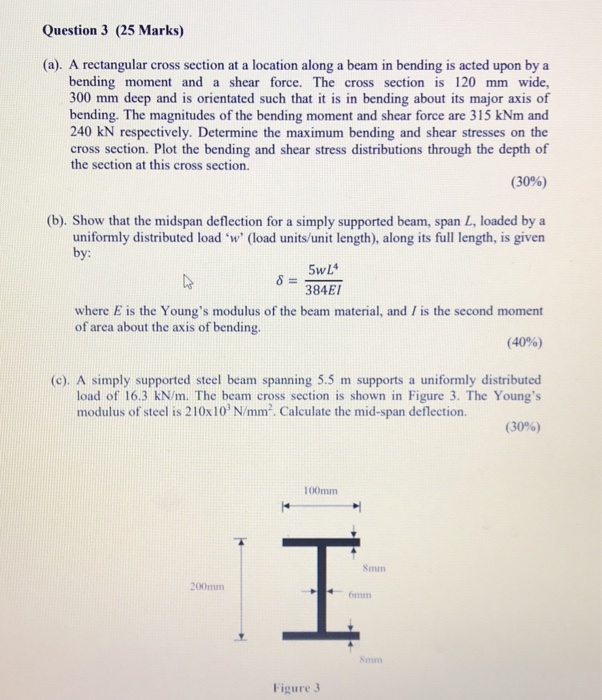Question 3 (25 Marks) (a). A rectangular cross section at a location along a beam in bending is acted upon by a bending moment and a shear force. The cross section is 120 mm wide, 300 mm deep and is orientated such that it is in bending about its major axis of bending. The magnitudes of the bending moment and shear force are 315 kNm and 240 kN respectively. Determine the maximum bending and shear stresses on the cross section....

• ### For the beam shown in the figure below Draw the shear and moment diagrams for this beam Calculate...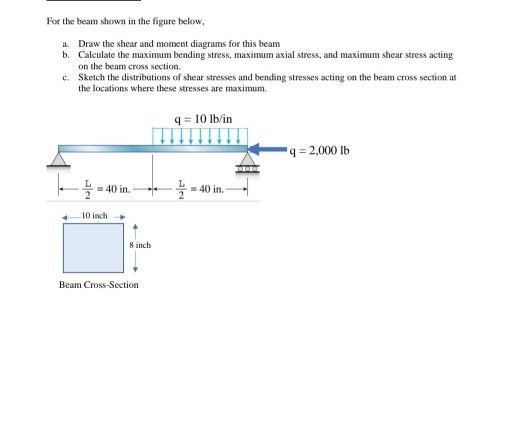For the beam shown in the figure below Draw the shear and moment diagrams for this beam Calculate the maximum bending stress, maximum axial stress, and maximum shear stress acting on the beam cross section Sketch the distributions of shear stresses and bending stresses acting on the beam cross section at the locations where these stresses are maximum. a. b. c. q 10 lb/in q 2,000 Ib 느-40 in ←_ 10 inch Beam Cross-Section For the beam shown in the...

• ### A concentrated force P is applied in the middle of a simply supported beam with the T-shaped cross section shown. The m...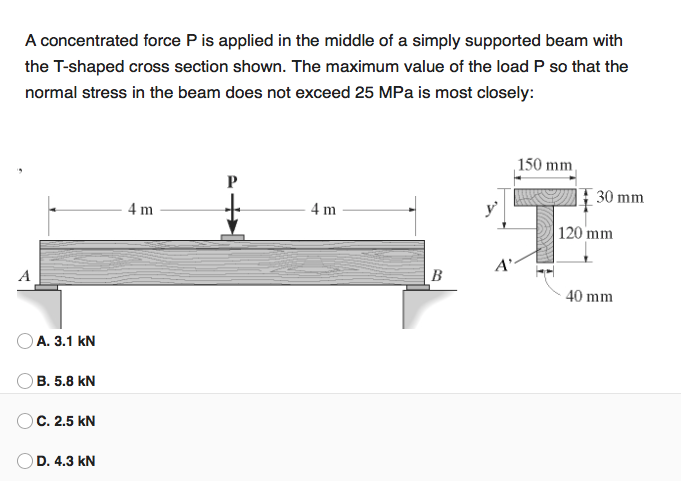A concentrated force P is applied in the middle of a simply supported beam with the T-shaped cross section shown. The maximum value of the load P so that the normal stress in the beam does not exceed 25 MPa is most closely: 150 mm P 30 mm 4m 4m 120 mm A' B A 40 mm A. 3.1 kN B. 5.8 kN Oc. 2.5 kN D. 4.3 kN A concentrated force P is applied in the middle of a...

• ### Figure 3 shows a cross-section of a combined open and closed section beam with a thickness of 2 m...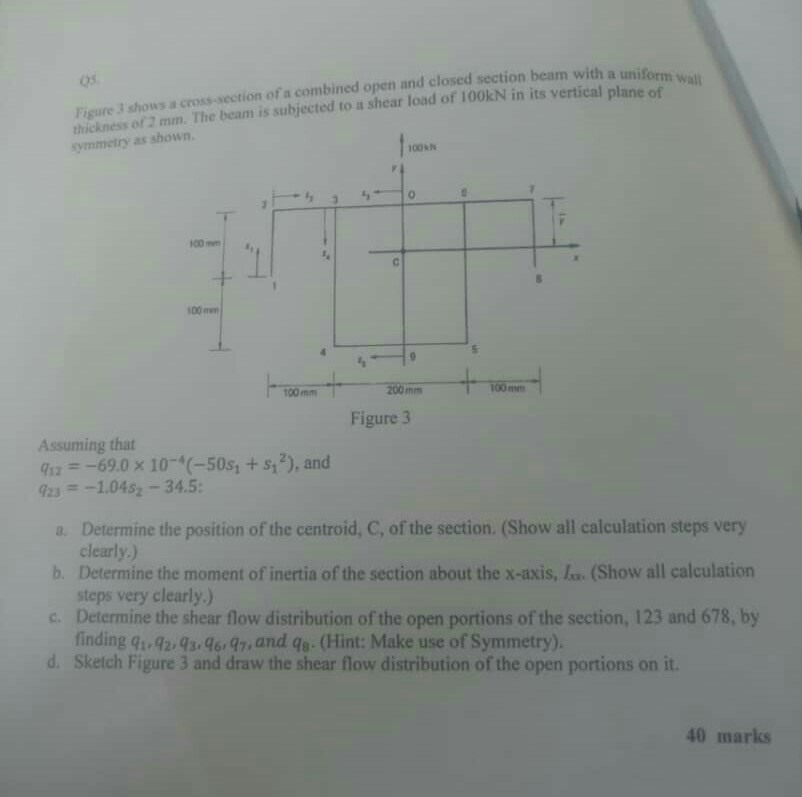Figure 3 shows a cross-section of a combined open and closed section beam with a thickness of 2 mm. The beam is subjected to a shear load of 100kN in its vertical plane ofh Wa symmetry as shown. 05 unif form wall 100kN 100mm 00mm 200 mm 100 Figure 3 Assuming that sz69.0 x 10-*(-50s, +s,2), and Determine the position of the centroid, C, of the section. (Show all calculation steps very a. b. Determine the moment of inertia of...

• ### The figure shown below is the cross section of a steel rectangular beam. The beam is subjected to transverse loading ap...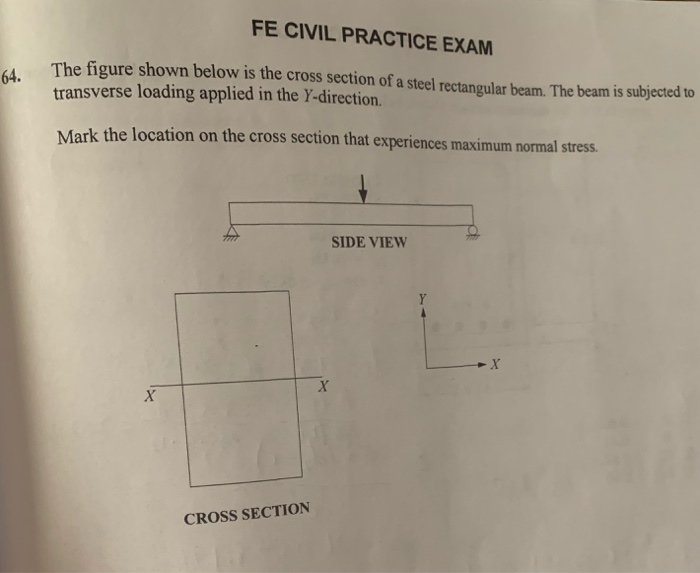The figure shown below is the cross section of a steel rectangular beam. The beam is subjected to transverse loading applied in the Y-direction. Mark the location on the cross section that experiences maximum normal stress. FE CIVIL PRACTICE EXAM The figure shown below is the cross section of a steel rectangular beam. The beam is subjected to 64. transverse loading applied in the Y-direction Mark the location on the cross section that experiences maximum normal stress SIDE VIEW CROSS...

• ### A beam with a cross section shown below is subjected to a positive moment about a horizontal axis...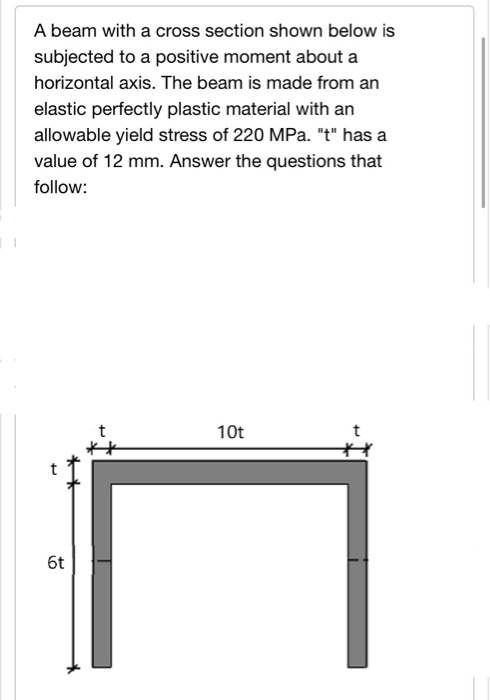A beam with a cross section shown below is subjected to a positive moment about a horizontal axis. The beam is made from an elastic perfectly plastic material with an allowable yield stress of 220 MPa. "t" has a value of 12 mm. Answer the questions that follow: 10t 6t Determine the centroid of this section i.e.as measured from the bottom of the section in [mm) - Determine the moment of inertia about the elastic neutral axis in [mm4] Determine...

• ### The cantilevered beam shown below is a sandwich beam with a plastic core and aluminum alloy faces...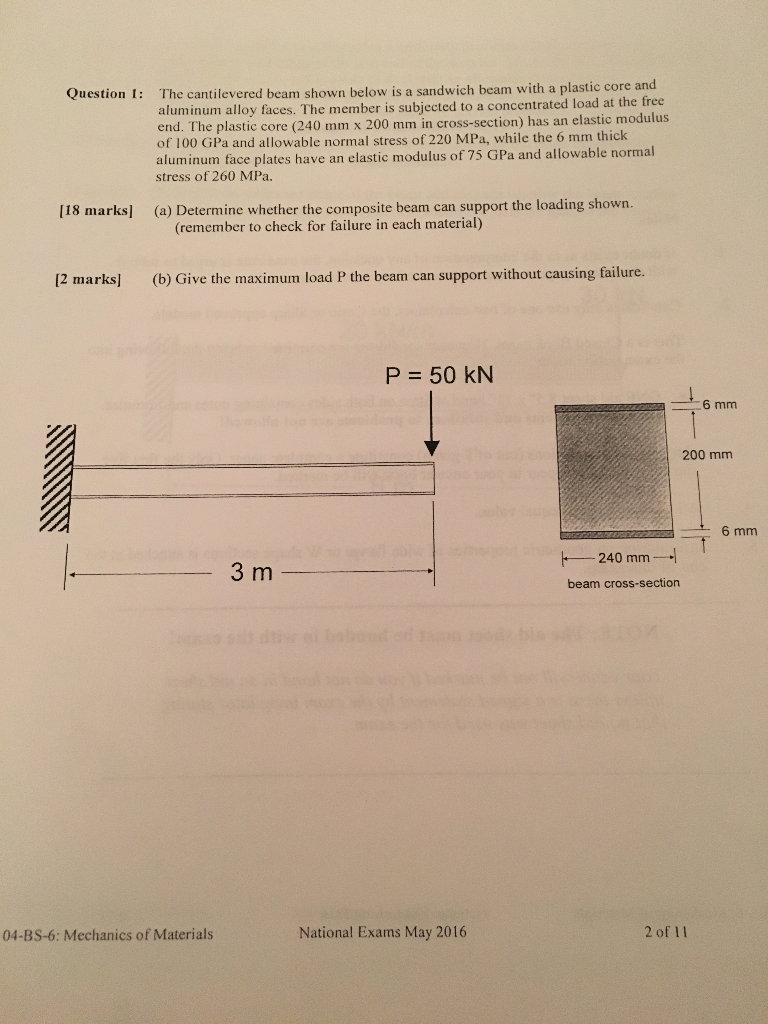The cantilevered beam shown below is a sandwich beam with a plastic core and aluminum alloy faces. The member is subjected to a concentrated load at the free end. The plastic core (240mm x 200 m m in cross section) has an elastic modulus of 100 GPa and allowable normal stress of 220 MPa, while the 6 mm thick aluminum face plates have an elastic modulus of 75 GPa and allowable normal stress of 260 MPa. Question I: 118 marks]...

• ### Question 4: Shear stress in a beam (40 marks) Consider the beam section illustrated in the figure to the right. The beam has a shearing force of V = 5 kN acting vertically through the shear center as...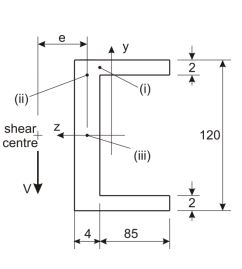Question 4: Shear stress in a beam (40 marks) Consider the beam section illustrated in the figure to the right. The beam has a shearing force of V = 5 kN acting vertically through the shear center as illustrated. (a) Determine the shear stress at the three points in the beam section labelled (i), (ii), and (iii). [20 marks] (b) Produce a plot showing the direction and distribution of shear stress across the beam section, noting the shape of the...

Need Online Homework Help?